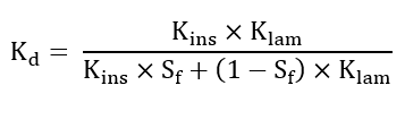# Lamination

## Laminations - Data

Here are the properties needed to define a new lamination:

 Category Label Unit Description Name * Family * Author * Manufacturer * Memo * Economic Price USD/kg Mechanical data Sheet thickness mm Stacking factor 1 Mass density kg/m3 Young modulus N/m2 Poisson ratio 1 Magnetic data Relative permeability 1 Magnetic polarization at saturation Js T Relative permeability 1 Knee coefficient 1 Iron Loss Hysteresis loss coefficient (kh) 1 Exponent of B for the hysteresis losses (αh) 1 Exponent of f for the hysteresis losses (βh) 1 Classical loss coefficient - Sine wave (kc x kαc) 1 Classical loss coefficient - Any wave (kc) 1 Exponent for the classical losses (αc) 1 Excess loss coefficient - Sine wave (ke x kαe) 1 Excess loss coefficient - Any wave (ke) 1 Exponent of B for the excess losses (αe) 1 Thermal data Reference temperature - Tref °C Thermal conductivity in the lamination direction at Tref W/K/m Thermal conductivity in the lamination insulation at Tref W/K/m Equivalent thermal conductivity in the lamination depth at Tref W/K/m Specific heat at Tref J/K/kg
Note: The B(H) curve is defined with an analytical model given in the Advanced section: Create a B(H) curve.
Note: A stacking factor is considered to define the B(H) curve to analyze the behavior of the magnetic circuit of the machine.

The user must define the magnetic characteristics of the solid material while the magnetic characteristics of the lamination stack are automatically deduced considering the value of the stacking factor.

See Advanced section: Create a B(H) curve.

Note: Electric properties are defined via iron loss model.
Note: Iron losses are defined with an analytical model given in Advanced section: Define iron loss parameters.
Note: The thermal conductivity “in depth” along the stacking direction: K d is computed as follows:
 S f Stacking factor K ins Thermal conductivity of the lamination insulation K lam Thermal conductivity in the laminationNote: The thermal conductivity of laminated regions is constant whatever is the temperature of the region.